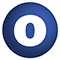# ValueOrdering

#### object ValueOrdering extends Ordering[Value]

An ordering by id for values of this set

Source
Enumeration.scala
Linear Supertypes
math.Ordering[Value], math.PartialOrdering[Value], math.Equiv[Value], Serializable, java.io.Serializable, Comparator[Value], AnyRef, Any
Ordering
1. Alphabetic
2. By inheritance
Inherited
1. ValueOrdering
2. Ordering
3. PartialOrdering
4. Equiv
5. Serializable
6. Serializable
7. Comparator
8. AnyRef
9. Any
1. Hide All
2. Show all
Visibility
1. Public
2. All

### Type Members

1. #### class Ops extends AnyRef

This inner class defines comparison operators available for `T`.

### Value Members

1. #### final def !=(arg0: AnyRef): Boolean

Definition Classes
AnyRef
2. #### final def !=(arg0: Any): Boolean

Definition Classes
Any
3. #### final def ##(): Int

Definition Classes
AnyRef → Any
4. #### final def ==(arg0: AnyRef): Boolean

Definition Classes
AnyRef
5. #### final def ==(arg0: Any): Boolean

Definition Classes
Any
6. #### final def asInstanceOf[T0]: T0

Definition Classes
Any
7. #### def clone(): AnyRef

Attributes
protected[java.lang]
Definition Classes
AnyRef
Annotations
( ... )
8. #### def compare(x: Value, y: Value): Int

Returns an integer whose sign communicates how x compares to y.

Returns an integer whose sign communicates how x compares to y.

The result sign has the following meaning:

• negative if x < y
• positive if x > y
• zero otherwise (if x == y)
Definition Classes
ValueOrderingOrdering → Comparator
9. #### final def eq(arg0: AnyRef): Boolean

Definition Classes
AnyRef
10. #### def equals(arg0: Any): Boolean

Definition Classes
AnyRef → Any
11. #### def equiv(x: Value, y: Value): Boolean

Return true if `x` == `y` in the ordering.

Return true if `x` == `y` in the ordering.

Definition Classes
OrderingPartialOrderingEquiv
12. #### def finalize(): Unit

Attributes
protected[java.lang]
Definition Classes
AnyRef
Annotations
( classOf[java.lang.Throwable] )
13. #### final def getClass(): Class[_]

Definition Classes
AnyRef → Any
14. #### def gt(x: Value, y: Value): Boolean

Return true if `x` > `y` in the ordering.

Return true if `x` > `y` in the ordering.

Definition Classes
OrderingPartialOrdering
15. #### def gteq(x: Value, y: Value): Boolean

Return true if `x` >= `y` in the ordering.

Return true if `x` >= `y` in the ordering.

Definition Classes
OrderingPartialOrdering
16. #### def hashCode(): Int

Definition Classes
AnyRef → Any
17. #### final def isInstanceOf[T0]: Boolean

Definition Classes
Any
18. #### def lt(x: Value, y: Value): Boolean

Return true if `x` < `y` in the ordering.

Return true if `x` < `y` in the ordering.

Definition Classes
OrderingPartialOrdering
19. #### def lteq(x: Value, y: Value): Boolean

Return true if `x` <= `y` in the ordering.

Return true if `x` <= `y` in the ordering.

Definition Classes
OrderingPartialOrdering
20. #### def max(x: Value, y: Value): Value

Return `x` if `x` >= `y`, otherwise `y`.

Return `x` if `x` >= `y`, otherwise `y`.

Definition Classes
Ordering
21. #### def min(x: Value, y: Value): Value

Return `x` if `x` <= `y`, otherwise `y`.

Return `x` if `x` <= `y`, otherwise `y`.

Definition Classes
Ordering
22. #### implicit def mkOrderingOps(lhs: Value): Ops

This implicit method augments `T` with the comparison operators defined in `scala.math.Ordering.Ops`.

This implicit method augments `T` with the comparison operators defined in `scala.math.Ordering.Ops`.

Definition Classes
Ordering
23. #### final def ne(arg0: AnyRef): Boolean

Definition Classes
AnyRef
24. #### final def notify(): Unit

Definition Classes
AnyRef
25. #### final def notifyAll(): Unit

Definition Classes
AnyRef
26. #### def on[U](f: (U) ⇒ Value): math.Ordering[U]

Given f, a function from U into T, creates an Ordering[U] whose compare function is equivalent to:

Given f, a function from U into T, creates an Ordering[U] whose compare function is equivalent to:

`def compare(x:U, y:U) = Ordering[T].compare(f(x), f(y))`
Definition Classes
Ordering
27. #### def reverse: math.Ordering[Value]

Return the opposite ordering of this one.

Return the opposite ordering of this one.

Definition Classes
OrderingPartialOrdering
28. #### final def synchronized[T0](arg0: ⇒ T0): T0

Definition Classes
AnyRef
29. #### def toString(): String

Definition Classes
AnyRef → Any
30. #### def tryCompare(x: Value, y: Value): Some[Int]

Returns whether a comparison between `x` and `y` is defined, and if so the result of `compare(x, y)`.

Returns whether a comparison between `x` and `y` is defined, and if so the result of `compare(x, y)`.

Definition Classes
OrderingPartialOrdering
31. #### final def wait(): Unit

Definition Classes
AnyRef
Annotations
( ... )
32. #### final def wait(arg0: Long, arg1: Int): Unit

Definition Classes
AnyRef
Annotations
( ... )
33. #### final def wait(arg0: Long): Unit

Definition Classes
AnyRef
Annotations
( ... )